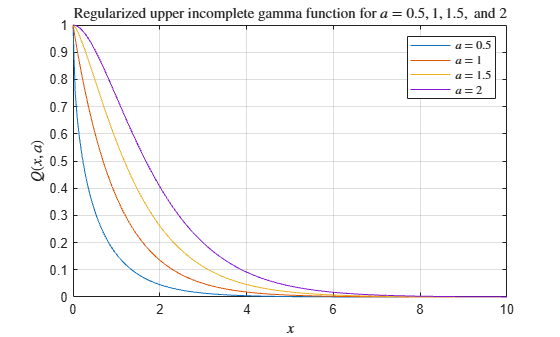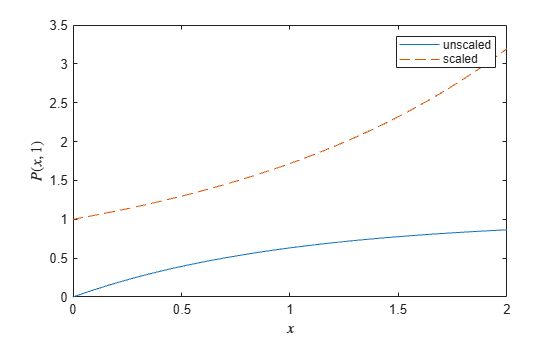gammainc

Incomplete gamma function

Description

example

Y = gammainc(X,A) returns the lower incomplete gamma function evaluated at the elements of X and A. Both X and A must be real, and A must be nonnegative.

example

Y = gammainc(X,A,type) returns the lower or upper incomplete gamma function. The choices for type are 'lower' (the default) and 'upper'.

example

Y = gammainc(X,A,scale) scales the resulting lower or upper incomplete gamma function to avoid underflow to zero or loss of accuracy. The choices for scale are 'scaledlower' and 'scaledupper'.

Examples

collapse all

Calculate the lower incomplete gamma function for $a$ = 0.5, 1, 1.5, and 2 within the interval $0\le x\le 10$. Loop over values of $a$, evaluate the function at each one, and assign each result to a column of Y.

A = [0.5 1 1.5 2];
X = 0:0.05:10;
Y = zeros(201,4);
for i = 1:4
Y(:,i) = gammainc(X,A(i));
end

Plot all of the functions in the same figure.

plot(X,Y)
grid on
legend('\$a = 0.5\$','\$a = 1\$','\$a = 1.5\$','\$a = 2\$','interpreter','latex')
title('Lower incomplete gamma function for \$a = 0.5, 1, 1.5,\$ and \$2\$','interpreter','latex')
xlabel('\$x\$','interpreter','latex')
ylabel('\$P(x,a)\$','interpreter','latex')Calculate the upper incomplete gamma function for $a$ = 0.5, 1, 1.5, and 2 within the interval $0\le x\le 10$. Loop over values of $a$, evaluate the function at each one, and assign each result to a column of Y.

A = [0.5 1 1.5 2];
X = 0:0.05:10;
Y = zeros(201,4);
for i = 1:4
Y(:,i) = gammainc(X,A(i),'upper');
end

Plot all of the functions in the same figure.

plot(X,Y)
grid on
legend('\$a = 0.5\$','\$a = 1\$','\$a = 1.5\$','\$a = 2\$','interpreter','latex');
title('Upper incomplete gamma function for \$a = 0.5, 1, 1.5,\$ and \$2\$','interpreter','latex')
xlabel('\$x\$','interpreter','latex')
ylabel('\$Q(x,a)\$','interpreter','latex')Calculate the unscaled lower incomplete gamma function and compare it to the scaled function.

Calculate the unscaled lower incomplete gamma function for $a=1$ within the interval $0\le x\le 2$. Plot the function.

a = 1;
x = 0:0.001:2;
Y = gammainc(x,a);
plot(x,Y);
xlabel('\$x\$','interpreter','latex');
ylabel('\$P(x,1)\$','interpreter','latex')
hold on

Next, calculate the scaled lower incomplete gamma function. Plot the function on the same graph. The scaled function has different asymptotic behavior near 0, which avoids underflow when $x$ is close to 0.

Ys = gammainc(x,a,'scaledlower');
plot(x,Ys,'--');
legend('unscaled','scaled')Input Arguments

collapse all

Input array, specified as a scalar, vector, matrix, or multidimensional array. The elements of X must be real. X and A must be the same size, or else one of them must be a scalar.

Data Types: single | double

Input array, specified as a scalar, vector, matrix, or multidimensional array. The elements of A must be real and nonnegative. X and A must be the same size, or else one of them must be a scalar.

Data Types: single | double

Type of incomplete gamma function, specified as 'lower' or 'upper'. If type is 'lower', then gammainc returns the lower incomplete gamma function. If type is 'upper', then gammainc returns the upper incomplete gamma function.

Scaling option, specified as 'scaledlower' or 'scaledupper'. If scale is 'scaledlower' or 'scaledupper', then gammainc scales the lower or upper incomplete gamma function by a factor of $\Gamma \left(a+1\right){e}^{x}/{x}^{a}$, where $\Gamma \left(a\right)$ is the gamma function. This scaling cancels out the asymptotic behavior of the function near 0, which avoids underflow with small arguments.

Limitations

• When x is negative, the incomplete gamma function can be inaccurate for abs(x) > a+1.

collapse all

Incomplete Gamma Function

The lower incomplete gamma function P and the upper incomplete gamma function Q are defined by

$\begin{array}{l}P\left(x,a\right)=\frac{1}{\Gamma \left(a\right)}{\int }_{0}^{x}{t}^{a-1}{e}^{-t}dt,\\ Q\left(x,a\right)=\frac{1}{\Gamma \left(a\right)}{\int }_{x}^{\infty }{t}^{a-1}{e}^{-t}dt.\end{array}$

The gamma function $\Gamma \left(a\right)$ is defined by

$\Gamma \left(a\right)={\int }_{0}^{\infty }{t}^{a-1}{e}^{-t}dt.$

MATLAB® uses the normalized definition of the incomplete gamma function, where $P\left(x,a\right)+Q\left(x,a\right)=1$.

The scaled lower and upper incomplete gamma function are defined by

$\begin{array}{l}{P}_{s}\left(x,a\right)=\frac{\Gamma \left(a+1\right)}{\Gamma \left(a\right)}\frac{{e}^{x}}{{x}^{a}}{\int }_{0}^{x}{t}^{a-1}{e}^{-t}dt,\\ {Q}_{s}\left(x,a\right)=\frac{\Gamma \left(a+1\right)}{\Gamma \left(a\right)}\frac{{e}^{x}}{{x}^{a}}{\int }_{x}^{\infty }{t}^{a-1}{e}^{-t}dt.\end{array}$

Some properties of the lower incomplete gamma function are:

• $\underset{x\to \infty }{\mathrm{lim}}P\left(x,a\right)=1\text{ }\text{for}\text{\hspace{0.17em}}a\ge 0$

• $\underset{x,a\to 0}{\mathrm{lim}}P\left(x,a\right)=1$

Tips

• When the upper incomplete gamma function is close to 0, specifying the 'upper' option to calculate the function is more accurate than subtracting the lower incomplete gamma function from 1.

 Olver, F. W. J., A. B. Olde Daalhuis, D. W. Lozier, B. I. Schneider, R. F. Boisvert, C. W. Clark, B. R. Miller, and B. V. Saunders, eds., Chapter 8. Incomplete Gamma and Related Functions, NIST Digital Library of Mathematical Functions, Release 1.0.22, Mar. 15, 2018.